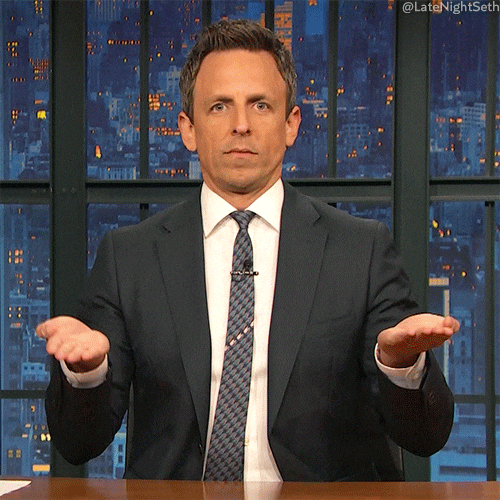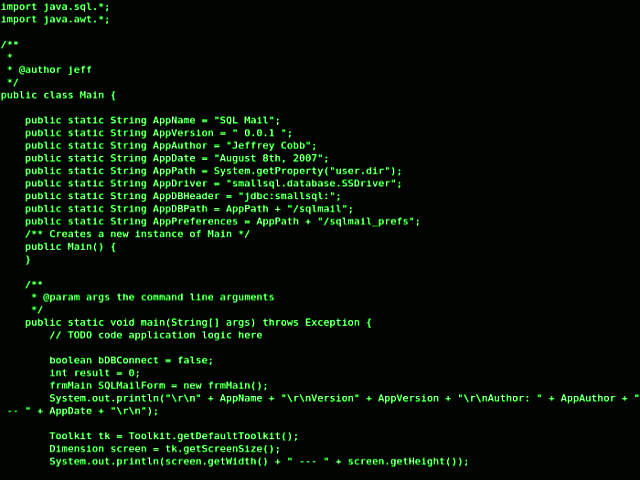# True or False?There will be a lot of new things in this chapter, but it shouldn't be very difficult: after all, in general, everything revolves around a simple idea - true or false?

Until now, we have always dealt only with primitive data types - with numbers and strings. Have you come across the term "primitive" in programming before? If not, I'll explain: "primitive" (they also say "simple") means that this data type is not an object (we'll come back to this point) and does not have built-in methods of work (that is, functions⚙️).The data type that you will definitely need is called boolean, or boolean. Boolean type always has the value either `true` ✅ - true, or` false` ❎ - false. And only this way, and nothing else! He is either lying or telling the truth - pan or disappear, the light is on or off, or there is or not. You either did your homework or you didn't. Only two 2️⃣ values are `true` ✅ or` false`.

## Equality operators​Boolean values come in handy when we need to compare something in JavaScript. When the need arises, we immediately call the comparison operators. Now we will sequentially study all eight comparison operators, but the thing is that as a result of each of them, we do not care we will always be left with a boolean value - either `true` ✅ or `false` ❎.

### Equals `==`​The equals operator first converts the operands to the same type, and then applies strict comparison. If both operands are objects, then JavaScript compares internal references that are equal if they refer to the same object in memory.

Syntax📖:

``x == y``

Examples:

``1 == 1 // true'1' == 1 // true1 == '1' // true3 == 5 // false0 == false // true'foo' == 'bar' // false``

Enter the examples one by one into the 🔔 `bool` variable of our `LIVE EDITOR`

Live Editor
Result

### Not equal to `!=`​The not equal operator returns🔄 `true` ✅ if the operands are not equal. It is similar to the equality operator, converting the operands to the same type before comparing. If both operands are objects, JavaScript compares internal references that are not equal if they refer to different objects in memory.

Syntax📖:

``x != y``

Examples:

``1! = 2 // true1! = '1' // false1! = '1' // false1! = True // false0! = False // false'foo'! = 'bar' // true``

Enter the examples one by one into the 🔔 `bool` variable of our `LIVE EDITOR`

Live Editor
Result

### Strictly equal to `===`​The operator returns true if the operands are strictly equal. Unlike the equals operator, this operator does not cast operands to the same type.

Syntax📖:

``x === y``

Examples:

``3 === 3 // true3 === '3' // false'foo' === 'foo' // true``

The operator makes sure that both the value and the type are strictly identical. In the case of `3 === '3'`, the value is, of course, identical, but the type is not: the first is a number, and the second is a string.

Enter the examples one by one into the 🔔 `bool` variable of our `LIVE EDITOR`

Live Editor
Result

### Strictly not equal to `! ==`​The strictly not equal operator returns true if the operands are not equal or their types differ from each other.

Syntax📖:

``x !== y``

Examples:

``3 !== '3' // true4 !== 3 // true``

Enter the examples one by one into the 🔔 `bool` variable of our `LIVE EDITOR`

Live Editor
Result

Why not use `==` and `! =`? But because, in general, there is never such a need. Whenever you can use them, you can always use both the strict `===` and `! ==`. If you want more flexibility in the answer (say, so that both `1` and `'1'` or `true` are equally accepted ✅), then you can simply include the desired answer options in the code itself (without changing this `===`).

##### Just understand the rule

Never use `==` or `! =`

## Comparison Operators​

### More `>`​The more operator returns true if the value of the left operand is greater than that of the right one.

Syntax📖:

``x > y``

Examples:

``4 > 3 // true1 > 5 // false``

Enter the examples one by one into the variable 🔔 `bool` in `LIVE EDITOR`

Live Editor
Result

### Less `<`​The less than operator returns true if the value of the operand on the left is less than the value of the operand on the right.

Syntax📖:

``x < y``

Examples:

``3 < 4 // true5 < 2 // false``

Enter the examples one by one into the 🔔 `bool` variable of our `LIVE EDITOR`

Live Editor
Result

### Greater than or equal to `>=`​The operator is greater than or equal to, returns true if the value of the operand on the left is greater than or equal to the value of the operand on the right.

Syntax📖:

``x >= y``

Examples:

``4 >= 3 // true3 >= 3 // true``

Enter the examples in the 🔔 `bool` variable one by one:

Live Editor
Result

### Less than or equal to `<=`​The operator is less than or equal, returns true if the value of the operand on the left is less than or equal to the value of the operand on the right.

Syntax📖:

``x <= y``

Examples:

``3 <= 4 // true3 <= 3 // true``

Enter the examples in the 🔔 `bool` variable one by one:

Live Editor
Result

## Conditional constructs​You must be thinking, "Well, all this boolean logic thing was very simple ... They are probably pretty useless and not used often." No matter how it is! Boolean values are used in programming more than all the time and most often in the form of conditionals (or expressions).

### What is a "conditional"?​Good question! A conditional is a clause that is used to run certain blocks of code📟 according to a given condition. The condition (for example, when comparing `x === y`) always returns a boolean value - either` true` ✅ or `false` ❎. Accordingly, if the value is `true` ✅, then the code should be run, otherwise the code block should be skipped. Let's look at some examples.

### Conditional Expressions with `if`​The `if` construction executes` instruction1`, if the condition is `true` ✅, if the condition is` false` ❎, then `instruction2` is executed.

Syntax📖:

``if (condition) {  instructions1} else {  instructions2}``

`condition` - An expression that is either true or false.

`instruction1` - An instruction executed if the value of `condition` is true ✅. Can be any statement, including a nested `if`. An empty statement can be used when no action is required.

`instruction2` - An instruction to execute if the value of `condition` is false. Can be any statement, including a nested `if`. Instructions can also be grouped into a block. Change the year in the 🔔 `whatIsTheYearNow` variable and note the output.

Live Editor
Result

### `if` not only with boolean values​Conditional expressions can work not only with boolean values, that is, with those that are not exactly `true` ✅ or` false` ❎ So, in general, we can safely use them in parentheses, as well as boolean values.

• All integers, except zero - `true`
• A string with at least one `true` character ✅
• An empty string is `false`

Let's try it, enter values into the 🔔 `bool` 👇 variable:

Live Editor
Result

### Comparison Operators in `if` Expressions​So far we have dealt with comparisons or conditionals with `if`, but so far we have not used them together, and they are just made for each other!

Live Editor
Result

### Multiple `else if` conditions​

Sometimes, you need to check several variants of a condition. This is done using the `else if` block. Change the year and see the output.

Live Editor
Result

### Conditional (ternary) operator `?`​The only JavaScript operator that accepts three operands: `condition` followed by a question mark `?`, Then `expression`, which is executed if the condition is true, followed by a colon `:`, and finally, `expression` which is executed if the condition is false. It is often used as a shorthand for an `if` statement.

Syntax📖:

``condition ? expression1 : expression2``

Parameters:

`condition` - An expression that takes the value `true` ✅ or `false` ❎.

`expression1`,` expression2` - Expressions whose values can be of any type.

Example 👇:

Live Editor
Result

## Problems?​Write to Discord chat.

## Questions:​What syntax is used in the equal operator?

1. `x == y`
2. `x = y`
3. `x - y`

In which case does the not equal operator return `true`?

1. If the operands are not equal
2. If the operands are equal
3. If both operands are objects

How is the operator equal to different from strictly equal?

1. Strictly equal does not cast operands to the same type
2. Strictly equalizes operands to the same type
3. Strictly ensures that the value is identical, but the type is not

What is the syntax for the operator strictly not equal?

1. `!= `
2. `!== `
3. `==! `

In which case does the operator return more false?

1. If the value of the left operand is greater than that of the right
2. If the value of the right operand is greater than that of the left
3. If the values ​​of the operand are the same

What is the syntax for the operator greater than or equal?

1. `> = `
2. `> => `
3. `> <= `

In which example will the less than or equal operator return true?

1. `4 <= 5`
2. `5 <= 4`
3. `3 <= 2`

What is a condition?

1. Instruction
2. Expression
3. Value

Which block is used to check multiple variants of a condition?

1. `else if`
2. `if`
3. `for`

Which operator takes 3 operands?

1. Conditional (ternary) operator
2. Greater than or equal
3. Less than or equal

## Contributors ✨​

Thanks goes to these wonderful people (emoji key):Dmitriy Vasilev 📖💵Resoner2005🐛 🎨 🖋